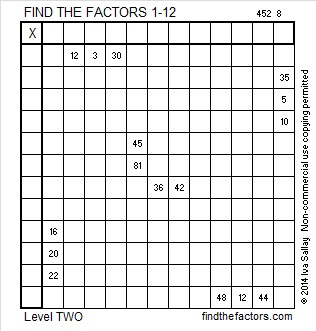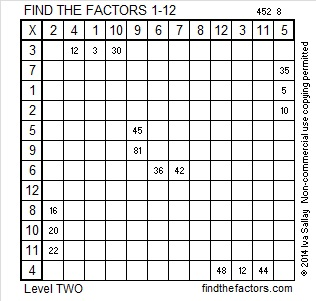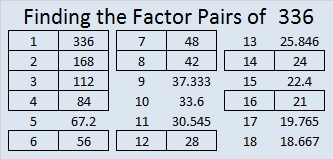# 336 and Level 2

Contents

### Today’s Puzzle:

The sixteen clues given in this puzzle are all you need to complete this multiplication table!Print the puzzles or type the factors on this excel file: 12 Factors 2014-12-29### Factors of 336:

• 336 is a composite number.
• Prime factorization: 336 = 2 x 2 x 2 x 2 x 3 x 7, which can be written 336 = (2^4) x 3 x 7
• The exponents in the prime factorization are 4, 1 and 1. Adding one to each and multiplying we get (4 + 1)(1 + 1)(1 + 1) = 5 x 2 x 2 = 20. Therefore 336 has exactly 20 factors.
• Factors of 336: 1, 2, 3, 4, 6, 7, 8, 12, 14, 16, 21, 24, 28, 42, 48, 56, 84, 112, 168, 336
• Factor pairs: 336 = 1 x 336, 2 x 168, 3 x 112, 4 x 84, 6 x 56, 7 x 48, 8 x 42, 12 x 28, 14 x 24 or 16 x 21
• Taking the factor pair with the largest square number factor, we get √336 = (√16)(√21) = 4√21 ≈ 18.330### Sum-Difference Puzzles:

84 has six factor pairs. One of those factor pairs adds up to 25, and another one subtracts to 25. Can you determine what those factor pairs are to solve the first puzzle below?

336 has ten factor pairs. One of them adds up to 50, and a different one subtracts to 50. If you can identify those factor pairs, then you can solve the second puzzle!The second puzzle is really just the first puzzle in disguise. Why would I say that?

## 2 thoughts on “336 and Level 2”

1.Joseph Nebus

Oh, I hope there’s something colorful you can do for New Year’s Eve and Day.

•ivasallay

I wasn’t planning on it, but I added some color to fulfill your request. Happy New Year!

This site uses Akismet to reduce spam. Learn how your comment data is processed.